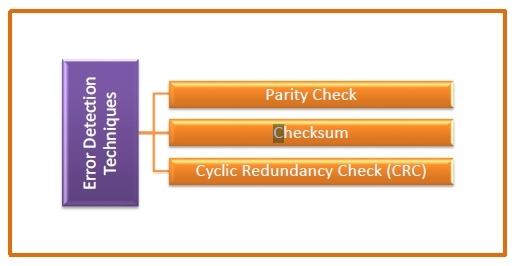# What are Error-Detecting Codes?

Error-detecting codes are a sequence of numbers generated by specific procedures for detecting errors in data that has been transmitted over computer networks.

When bits are transmitted over the computer network, they are subject to get corrupted due to interference and network problems. The corrupted bits leads to spurious data being received by the receiver and are called errors.

Error – detecting codes ensures messages to be encoded before they are sent over noisy channels. The encoding is done in a manner so that the decoder at the receiving end can detect whether there are errors in the incoming signal with high probability of success.

## Features of Error Detecting Codes

• Error detecting codes are adopted when backward error correction techniques are used for reliable data transmission. In this method, the receiver sends a feedback message to the sender to inform whether an error-free message has been received or not. If there are errors, then the sender retransmits the message.

• Error-detecting codes are usually block codes, where the message is divided into fixed-sized blocks of bits, to which redundant bits are added for error detection.

• Error detection involves checking whether any error has occurred or not. The number of error bits and the type of error does not matter.

## Error Detection Techniques

There are three main techniques for detecting errors## Parity Check

Parity check is done by adding an extra bit, called parity bit to the data to make number of 1s either even in case of even parity, or odd in case of odd parity.

While creating a frame, the sender counts the number of 1s in it and adds the parity bit in following way

• In case of even parity: If number of 1s is even then parity bit value is 0. If number of 1s is odd then parity bit value is 1.

• In case of odd parity: If number of 1s is odd then parity bit value is 0. If number of 1s is even then parity bit value is 1.

On receiving a frame, the receiver counts the number of 1s in it. In case of even parity check, if the count of 1s is even, the frame is accepted, otherwise it is rejected. Similar rule is adopted for odd parity check.

Parity check is suitable for single bit error detection only.

## Checksum

In this error detection scheme, the following procedure is applied

• Data is divided into fixed sized frames or segments.

• The sender adds the segments using 1’s complement arithmetic to get the sum. It then complements the sum to get the checksum and sends it along with the data frames.

• The receiver adds the incoming segments along with the checksum using 1’s complement arithmetic to get the sum and then complements it.

• If the result is zero, the received frames are accepted; otherwise they are discarded.

## Cyclic Redundancy Check (CRC)

Cyclic Redundancy Check (CRC) involves binary division of the data bits being sent by a predetermined divisor agreed upon by the communicating system. The divisor is generated using polynomials.

• Here, the sender performs binary division of the data segment by the divisor. It then appends the remainder called CRC bits to the end of data segment. This makes the resulting data unit exactly divisible by the divisor.

• The receiver divides the incoming data unit by the divisor. If there is no remainder, the data unit is assumed to be correct and is accepted. Otherwise, it is understood that the data is corrupted and is therefore rejected.